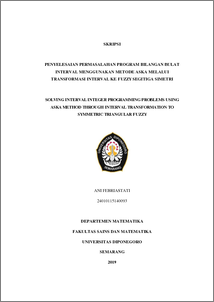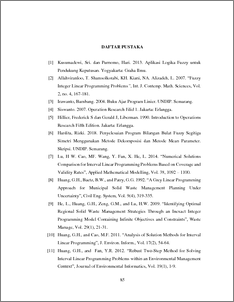# SOLVING INTERVAL INTEGER PROGRAMMING PROBLEMS USING ASKA METHOD THROUGH INTERVAL TRANSFORMATION TO SYMMETRIC TRIANGULAR FUZZY

FEBRIASTATI, ANI (2019) SOLVING INTERVAL INTEGER PROGRAMMING PROBLEMS USING ASKA METHOD THROUGH INTERVAL TRANSFORMATION TO SYMMETRIC TRIANGULAR FUZZY. Undergraduate thesis, UNDIP.Preview
PDF
190KbPDF
Restricted to Repository staff only

739KbPreview
PDF
62Kb

## Abstract

Linear programming is one of the models that can be used to solve optimization problem. In a real life, decision variable must be an integer, however in a linear programming the solution is not necessarily an integer. Therefore, we need an integer programming so that the decision variable is an integer. One of the assumptions used in the integer programming problem is deterministic. However, in a real life, these deterministic assumptions are difficult to fulfill. To resolve this problem, it can be approached by using a decision variable whose parameters are interval. An integer linear programming with interval parameters is called an interval integer linear programming (𝐼𝐼𝐿𝑃). 𝐼𝐼𝐿𝑃 problems can be solved using ASKA method. Firstly, the 𝐼𝐼𝐿𝑃 is transformed into a symmetric triangular fuzzy integer program (STFILP), then the STFILP is brought to two problems of firm integer linear programming (𝐼𝐿𝑃 crisp) where each 𝐼𝐼𝐿𝑃 will be solved using cutting plane method with fractional algorithm. The results of the 𝑆𝑇𝐹𝐼𝐿𝑃 ’s optimal solution obtained from the settlement using ASKA method and it is the optimal solutions from the 𝐼𝐼𝐿𝑃. Keyword: integer linear programming, interval integer linear programming, fuzzy integer linear programming, ASKA method.

Item Type: Thesis (Undergraduate) Q Science > QA Mathematics Faculty of Science and Mathematics > Department of Mathematics 84210 INVALID USER 11 Jun 2022 14:36 11 Jun 2022 14:36

Repository Staff Only: item control page